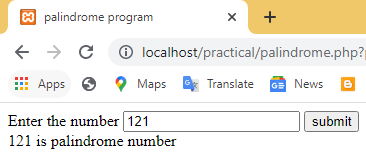# Palindrome program in PHP

In this program, You will learn how to check number is palindrome or not in php

a number is a palindrome if the reversed number is equal to the original number. for example 121, 101, 12321 are palindrome numbers.

``````
<!DOCTYPE html>
<html lang="en">
<title>palindrome program</title>
<body>
<form>
Enter the number <input type="number" name="palindrome">
<input type="submit" value="submit" name="click"></br>
</form>
</body>
</html>
<?php
if(isset(\$_GET["click"]))
{
\$n=\$_GET['palindrome'];
\$copy=\$n;
\$rev=0;
while(\$n>0)
{
/*It will find the reverse of the input entered by the user.*/
\$rev=\$rev*10+\$n%10;
\$n=(int)(\$n/10);
}
// Compare the reverse of input with the temporary variable
if(\$copy==\$rev)
{
echo \$copy.' is palindrome number';
}
else
{
echo \$copy.' is not palindrome number';
}
}
?>``````

## output### Logic to check whether input entered by the user is a palindrome number or not.

a step-by-step explanation of the palindrome number program given below:

1. In this program, you will take input from the user in the HTML input box and when the user clicks on submit button, the HTML form gets submitted, and PHP `if( isset(\$_GET["click"])` will evaluate the true expression.
2. Store the user input in a variable `n`. i.e `\$n =\$_GET['palindrome']`
3. Copy the user value i.e `n` into the `copy` variable for later comparison.
4. Iterate while loop until `n` value becomes zero.
5. reversed the number and stored the reverse number in the \$rev variable. `\$rev=\$rev*10+\$n%10;`
6. divide the value of `n` by 10 and after dividing store the integer value in the same variable `n`. ` \$n=(int)(\$n/10);`
7. After reversing the number check whether the reversed number is equal to the original number with the help of an if-statement.
8. If the reversed number is equal to the original number then if-statement will get executed it will print 'number is palindrome number'.
9. If the reversed number is not equal to the original number then else part will get executed and it will print 'number is not palindrome'.